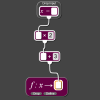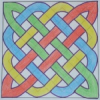# Resources tagged with: Factors and multiples

Filter by: Content type:
Age range:
Challenge level:

### There are 106 results

Broad Topics > Properties of Numbers > Factors and multiples### Take Three from Five

##### Age 14 to 16 Challenge Level:

Caroline and James pick sets of five numbers. Charlie chooses three of them that add together to make a multiple of three. Can they stop him?##### Age 11 to 14 Challenge Level:

Great Granddad is very proud of his telegram from the Queen congratulating him on his hundredth birthday and he has friends who are even older than he is... When was he born?### Hot Pursuit

##### Age 11 to 14 Challenge Level:

I added together the first 'n' positive integers and found that my answer was a 3 digit number in which all the digits were the same...### Cogs

##### Age 11 to 14 Challenge Level:

A and B are two interlocking cogwheels having p teeth and q teeth respectively. One tooth on B is painted red. Find the values of p and q for which the red tooth on B contacts every gap on the. . . .### DOTS Division

##### Age 14 to 16 Challenge Level:

Take any pair of two digit numbers x=ab and y=cd where, without loss of generality, ab > cd . Form two 4 digit numbers r=abcd and s=cdab and calculate: {r^2 - s^2} /{x^2 - y^2}.### Counting Factors

##### Age 11 to 14 Challenge Level:

Is there an efficient way to work out how many factors a large number has?### Hypotenuse Lattice Points

##### Age 14 to 16 Challenge Level:

The triangle OMN has vertices on the axes with whole number co-ordinates. How many points with whole number coordinates are there on the hypotenuse MN?### Three Times Seven

##### Age 11 to 14 Challenge Level:

A three digit number abc is always divisible by 7 when 2a+3b+c is divisible by 7. Why?### Even So

##### Age 11 to 14 Challenge Level:

Find some triples of whole numbers a, b and c such that a^2 + b^2 + c^2 is a multiple of 4. Is it necessarily the case that a, b and c must all be even? If so, can you explain why?### Special Sums and Products

##### Age 11 to 14 Challenge Level:

Find some examples of pairs of numbers such that their sum is a factor of their product. eg. 4 + 12 = 16 and 4 × 12 = 48 and 16 is a factor of 48.### Got It

##### Age 7 to 14 Challenge Level:

A game for two people, or play online. Given a target number, say 23, and a range of numbers to choose from, say 1-4, players take it in turns to add to the running total to hit their target.### LCM Sudoku II

##### Age 11 to 18 Challenge Level:

You are given the Lowest Common Multiples of sets of digits. Find the digits and then solve the Sudoku.### What Numbers Can We Make?

##### Age 11 to 14 Challenge Level:

Imagine we have four bags containing a large number of 1s, 4s, 7s and 10s. What numbers can we make?### What Numbers Can We Make Now?

##### Age 11 to 14 Challenge Level:

Imagine we have four bags containing numbers from a sequence. What numbers can we make now?### Got it for Two

##### Age 7 to 14 Challenge Level:

Got It game for an adult and child. How can you play so that you know you will always win?### Gabriel's Problem

##### Age 11 to 14 Challenge Level:

Gabriel multiplied together some numbers and then erased them. Can you figure out where each number was?##### Age 11 to 14 Challenge Level:

List any 3 numbers. It is always possible to find a subset of adjacent numbers that add up to a multiple of 3. Can you explain why and prove it?##### Age 7 to 14 Challenge Level:

I added together some of my neighbours' house numbers. Can you explain the patterns I noticed?### Summing Consecutive Numbers

##### Age 11 to 14 Challenge Level:

15 = 7 + 8 and 10 = 1 + 2 + 3 + 4. Can you say which numbers can be expressed as the sum of two or more consecutive integers?### Have You Got It?

##### Age 11 to 14 Challenge Level:

Can you explain the strategy for winning this game with any target?### N000ughty Thoughts

##### Age 14 to 16 Challenge Level:

How many noughts are at the end of these giant numbers?### Repeaters

##### Age 11 to 14 Challenge Level:

Choose any 3 digits and make a 6 digit number by repeating the 3 digits in the same order (e.g. 594594). Explain why whatever digits you choose the number will always be divisible by 7, 11 and 13.### Just Repeat

##### Age 11 to 14 Challenge Level:

Think of any three-digit number. Repeat the digits. The 6-digit number that you end up with is divisible by 91. Is this a coincidence?### Big Powers

##### Age 11 to 16 Challenge Level:

Three people chose this as a favourite problem. It is the sort of problem that needs thinking time - but once the connection is made it gives access to many similar ideas.### Two Much

##### Age 11 to 14 Challenge Level:

Explain why the arithmetic sequence 1, 14, 27, 40, ... contains many terms of the form 222...2 where only the digit 2 appears.### Inclusion Exclusion

##### Age 11 to 14 Challenge Level:

How many integers between 1 and 1200 are NOT multiples of any of the numbers 2, 3 or 5?### Can You Find a Perfect Number?

##### Age 7 to 14### What a Joke

##### Age 14 to 16 Challenge Level:

Each letter represents a different positive digit AHHAAH / JOKE = HA What are the values of each of the letters?### Cuboids

##### Age 11 to 14 Challenge Level:

Can you find a cuboid that has a surface area of exactly 100 square units. Is there more than one? Can you find them all?### Data Chunks

##### Age 14 to 16 Challenge Level:

Data is sent in chunks of two different sizes - a yellow chunk has 5 characters and a blue chunk has 9 characters. A data slot of size 31 cannot be exactly filled with a combination of yellow and. . . .### Star Product Sudoku

##### Age 11 to 16 Challenge Level:

The puzzle can be solved by finding the values of the unknown digits (all indicated by asterisks) in the squares of the $9\times9$ grid.### Shifting Times Tables

##### Age 11 to 14 Challenge Level:

Can you find a way to identify times tables after they have been shifted up or down?### Dozens

##### Age 7 to 14 Challenge Level:

Do you know a quick way to check if a number is a multiple of two? How about three, four or six?### Mathematical Swimmer

##### Age 11 to 14 Challenge Level:

Can you work out how many lengths I swim each day?### Counting Cogs

##### Age 7 to 14 Challenge Level:

Which pairs of cogs let the coloured tooth touch every tooth on the other cog? Which pairs do not let this happen? Why?### Exploring Simple Mappings

##### Age 11 to 14 Challenge Level:

Explore the relationship between simple linear functions and their graphs.### Drawing Celtic Knots

##### Age 11 to 14 Challenge Level:

Here is a chance to create some Celtic knots and explore the mathematics behind them.### Factor Track

##### Age 7 to 14 Challenge Level:

Factor track is not a race but a game of skill. The idea is to go round the track in as few moves as possible, keeping to the rules.### A Biggy

##### Age 14 to 16 Challenge Level:

Find the smallest positive integer N such that N/2 is a perfect cube, N/3 is a perfect fifth power and N/5 is a perfect seventh power.### Number Families

##### Age 11 to 14 Challenge Level:

How many different number families can you find?### Common Divisor

##### Age 14 to 16 Challenge Level:

Find the largest integer which divides every member of the following sequence: 1^5-1, 2^5-2, 3^5-3, ... n^5-n.### Satisfying Statements

##### Age 11 to 14 Challenge Level:

Can you find any two-digit numbers that satisfy all of these statements?### Mod 3

##### Age 14 to 16 Challenge Level:

Prove that if a^2+b^2 is a multiple of 3 then both a and b are multiples of 3.### Beelines

##### Age 14 to 16 Challenge Level:

Is there a relationship between the coordinates of the endpoints of a line and the number of grid squares it crosses?### Diagonal Product Sudoku

##### Age 11 to 16 Challenge Level:

Given the products of diagonally opposite cells - can you complete this Sudoku?##### Age 11 to 14 Challenge Level:

Make a set of numbers that use all the digits from 1 to 9, once and once only. Add them up. The result is divisible by 9. Add each of the digits in the new number. What is their sum? Now try some. . . .### Really Mr. Bond

##### Age 14 to 16 Challenge Level:

115^2 = (110 x 120) + 25, that is 13225 895^2 = (890 x 900) + 25, that is 801025 Can you explain what is happening and generalise?### Remainder

##### Age 11 to 14 Challenge Level:

What is the remainder when 2^2002 is divided by 7? What happens with different powers of 2?### Divisibility Tests

##### Age 11 to 16